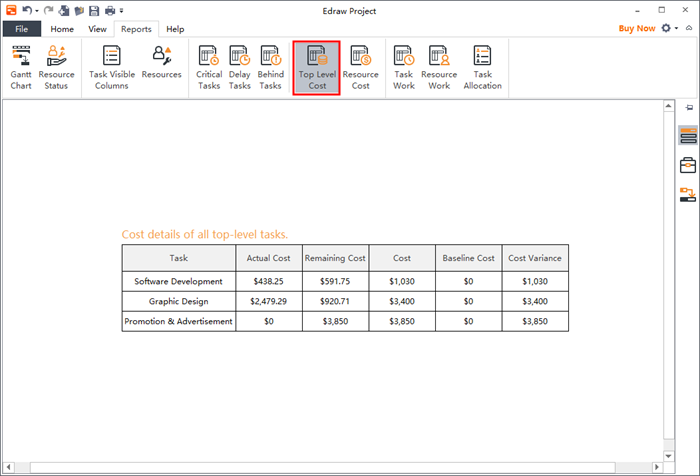Edraw Support Center

Get quick answers and step-by-step instructions in our support center.

Edraw Project Guide

• Video Tutorial

Edraw Project Video

Top Level Cost

In the Top Level Cost report, types of cost in a project life cycle includes:

• Actual Cost: Costs that have been incurred for tasks, resources or assignments.
• Remaining Cost: Difference between baseline cost and actual cost.
• Cost: When the tasks are planned and the pay rates of resources in a project are settled, Edraw Project will calculate the scheduled cost of a task or a resource.
• Baseline Cost: Rough estimate cost proposed by the project manager.
• Cost Variance: Difference between the scheduled cost and baseline cost.

The following are formulas to calculate different types of cost:

• Actual Cost = (Cost - Cost Per Use) * Progress + Cost Per Use
• Remaining Cost = Cost - Actual Cost
• Actual Cost = Actual Work*Standard Rate*Cost Per Use